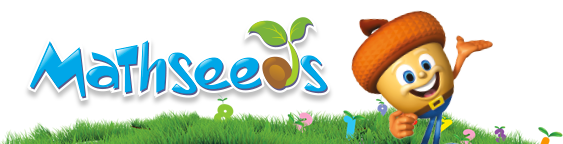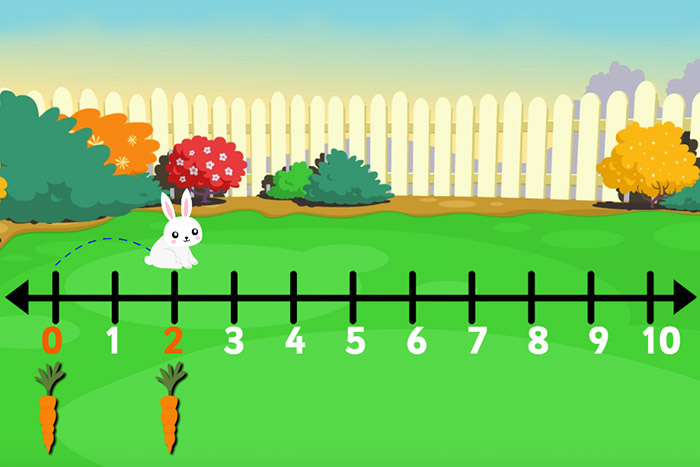## Start your FREE trial today!

+1 877 467 6851 customerservice@3plearning.caBack to ArticlesFirst grade math is a significant year for young learners, who will start to build on the basic mathematical knowledge learned in kindergarten. By first grade, students should be able to identify basic shapes, describe measurable attributes such as length, width, and height, and understand the concepts of addition ("putting together") and subtraction ("taking away").

There are some big differences between kindergarten and first grade math standards. Students at a first grade level are expected to move beyond a mere understanding of numbers and know how to independently reason with numbers.

In first grade, children are introduced to time, money, and the meaning of numbers greater than those they can count. First graders begin solving simple addition and subtraction problems. They learn to skip count by 2s, 5s, and 10s—a skill that will help them later when solving math equations. They also begin to work with 2- and 3‑dimensional geometric shapes.

First grade math students are expected to be able to measure length, tell time, and represent and interpret data. Additionally, they should be able to use place value concepts to add and subtract, and know how to represent and solve simple addition and subtraction problems.The Mathseeds program fully covers grades K–3 math skills, featuring 50 lessons per grade level.

Lessons 51–100 feature Mango the monkey and friends as they introduce and explore different math concepts. Children learn to count to 100, order numbers, and identify ordinal numbers. They develop an understanding of place value including regrouping, and also practice their subtraction skills.

Students learn how to add and subtract to 10, and then within 100. Strategies include counting on, counting back, near doubles, and using number fact families. They learn how to skip count by 2s, 5s, and 10s, as well as the early multiplication and division skills of grouping and sharing.

Later, students learn to identify bills and coins, and use addition to find amounts of money. They explore fractions, focusing on wholes, halves, and fourths. Students continue to investigate the features of 2D shapes and 3D objects. They follow simple directions to a particular location and learn to read clocks to the half‑hour. They also work with early chance concepts, tally charts, and simple picture graphs.

### Lesson Number and Name

51 Addition to 10 with Two and Three groups
52 Sorting and Grouping 2D Shapes
53 Subtraction 1
54 O'clock
55 Near and Far
56 Subtraction 2
57 Position 1
58 Subtraction on a Number Line
59 Area
60 Counting 20-30
61 Wholes and Halves
62 Sorting and Grouping 3D Objects
63 Ordinal Numbers
64 Money
66 Halves and Quarters
67 Counting 30-40

### Lesson Number and Name

68 Find the Difference 1
69 Putting Shapes Together
70 O'clock & Half Past
71 Sharing 1
72 Doubles to Double 10
73 Mass
74 Grouping
75 Counting 40-50
76 The Equal Sign
77 Skip Counting by 2s & 5s
78 Position 2
79 Counting by 10s
80 Data 1
81 Counting 50-70
82 Chance 1
83 Money 2
84 Measuring Length

### Lesson Number and Name

85 Find the Difference 2
86 Counting 70-100
87 Half Past and Digital Time
89 Capacity 2
90 Skip Counting
91 Near Doubles to 20
92 Change from \$20
93 Number Fact Families
94 Position 3
96 Bridging to Ten
97 Data 2
99 3D Objects
100 Subtracting Unknown Numbers

## 1st Grade Math and Provincial Standards

The Mathseeds lessons provide comprehensive coverage of K–3 Math provincial standards

The correlation charts here clearly show the scope and range of the Mathseeds lessons across all K–3 math standards, content, and skills.

Teachers can use these charts to identify the ways in which Mathseeds lessons meet the learning objectives and standards for your first grade math students.

## 1st Grade Math Worksheets and Lesson Plans

Mathseeds features a comprehensive range of teacher resources, including first grade worksheets, lesson plans, and assessment material. There is a set of teacher resources to match each of the 200 lessons in the program. View some sample worksheets here.

The Teacher Toolkit in Mathseeds also features math posters to brighten up the classroom, digital big books for each grade level, and much more.

Are you new to Mathseeds? Start a free trial here of the award-winning online math program for students in grades K–3.